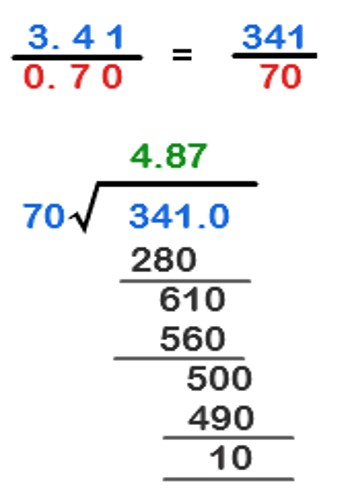Division of Decimals by Decimals

The procedure for the division of decimals is very similar to the division of whole numbers. Make the divisor into a whole number by multiplying both it and the dividend by the same number (such as 10, 100, 1000 etc.). An easy way to do this is to move the decimal point to the right end of the divisor and move the decimal point of the dividend the same number of places.

To divide a decimal by another decimal:

• Move the decimal point in the divisor to the right until it is a whole number.
• Move the decimal point in the dividend to the right by the same number of places as the decimal point was moved to make the divisor a whole number.
• Then divide the new dividend by the new divisor.How to divide a four digit decimal number by a two digit decimal number (e.g 0.4131 ÷ 0.17).

Place the divisor before the division bracket and place the dividend (0.4131) under it

0.17)0.4131

Multiply both the divisor and dividend by 100 so that the divisor is not a decimal but a whole number. In other words move the decimal point two places to the right in both the divisor and dividend

17)41.31

Proceed with the division as you normally would except put the decimal point in the answer or quotient exactly above where it occurs in the dividend. For example:

2.43

17)41.31

Here follow:

• If the divisor is not a whole number, move decimal point to right to make it a whole number and move decimal point in dividend the same number of places.
• Divide as usual. Keep dividing until the answer terminates or repeats.
• Put decimal point directly above decimal point in the dividend.

Information Source: